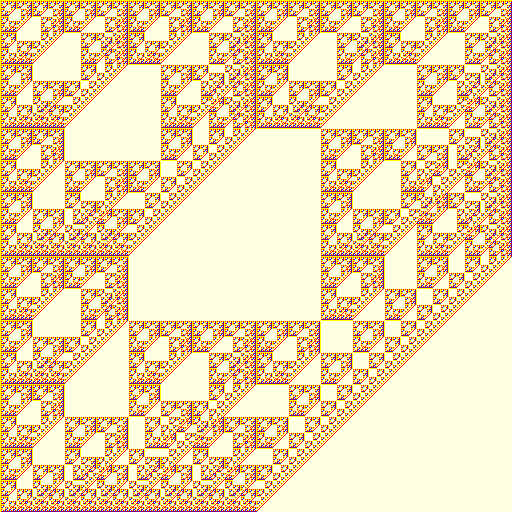## Diamond

### Info

Denote the elements of the field $F_{4}$ by $\{0, 1, w, w + 1\}$, where w satisfies the following equation with coefficients in $F_{2}: w^{2} + w + 1 = 0$. Diamond is a recurrent double sequence defined by $a(i, 0) = a(0, j) = 1$ and $a(i, j) = f(a(i, j-1), a(i-1, j-1), a(i-1, j))$, where $f(x, y, z) = w x^{2} + y + (w + 1) y^{2} + w z^{2}$. This recurrent double sequence can be also obtained using a system of substitutions of type 4 -> 8 with 42 rules.

The system of substitutions is too large to be presented here.

### Patch